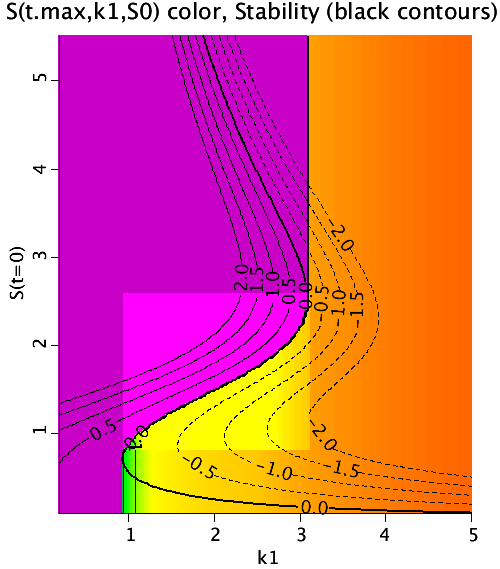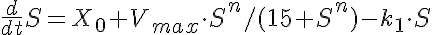# Cooperativity

Model number
0342

This cooperativity binding model exhibits bistable bifurcation.Figure 1: Cooperativity equation with bistable bifurcation.

## Description

```This is a model of binding cooperativity which is bistable.
The differential equation for S is a function of
X0, Vmax, n, and k1, and t, with initial value S0.

The goal is to explore the stability as a function of
k1 and S0, for fixed values of X0, Vmax, and n.

The realDomain values are defined for t (time) k1 and S0
(the initial value of S). The final value of S (S(t.max,k1,S0)
is contoured in color. Notice the sharp demarcation between the
purple region and orange region where a small shift in k1 and S0
give very different final values.

The plot also shows the stability line (where the
ODE does not change value (dark contour labelled 0.)
However between approximately 0.75 < S < 2.5
the smallest displacements from the stability line
piece of the stability line is a bifurcation.  Along
the rest of the dS/dt=0 curve, small perturbations in k1
and S0 cause only small perturbations in the steady state
solutions.

The line where dS/dt=0 is given as

X0+Vmax*S^n/(15+S^n) - k1*S = 0.

Setting k1 =2, X0 = 1/2, Vmax = 10, and n=4, we have a
quintic equation. Solving for S, there are 3 real roots and
two complex roots. The real roots occur at
S= 0.251329652519894, 1.49225862823290, 5.14522788409661.

The unstable point is at S=1.4922586282329 and this is shown
and inspecting the Bistability plot page. S0 = 1.491 and 1.492
have a steady state value = 0.2513965, whereas S0 = 1.493 and
1.494 have a steady state value of 5.1452279. These two different
final values are the other two real roots when solving dS/dt=0.
```

## Equations

#### Cooperativity equationThe equations for this model may be viewed by running the JSim model applet and clicking on the Source tab at the bottom left of JSim's Run Time graphical user interface. The equations are written in JSim's Mathematical Modeling Language (MML). See the Introduction to MML and the MML Reference Manual. Additional documentation for MML can be found by using the search option at the Physiome home page.

References

None yet.

Key terms
cooperativity
bistable
bistability
bifurcation
Acknowledgements

Please cite https://www.imagwiki.nibib.nih.gov/physiome in any publication for which this software is used and send one reprint to the address given below:
The National Simulation Resource, Director J. B. Bassingthwaighte, Department of Bioengineering, University of Washington, Seattle WA 98195-5061.

Model development and archiving support at https://www.imagwiki.nibib.nih.gov/physiome provided by the following grants: NIH U01HL122199 Analyzing the Cardiac Power Grid, 09/15/2015 - 05/31/2020, NIH/NIBIB BE08407 Software Integration, JSim and SBW 6/1/09-5/31/13; NIH/NHLBI T15 HL88516-01 Modeling for Heart, Lung and Blood: From Cell to Organ, 4/1/07-3/31/11; NSF BES-0506477 Adaptive Multi-Scale Model Simulation, 8/15/05-7/31/08; NIH/NHLBI R01 HL073598 Core 3: 3D Imaging and Computer Modeling of the Respiratory Tract, 9/1/04-8/31/09; as well as prior support from NIH/NCRR P41 RR01243 Simulation Resource in Circulatory Mass Transport and Exchange, 12/1/1980-11/30/01 and NIH/NIBIB R01 EB001973 JSim: A Simulation Analysis Platform, 3/1/02-2/28/07.